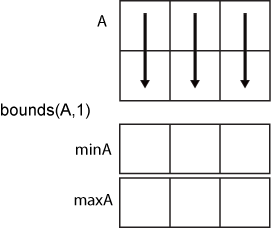# bounds

## 语法

``[minA,maxA] = bounds(A)``
``[minA,maxA] = bounds(A,'all')``
``[minA,maxA] = bounds(A,dim)``
``[minA,maxA] = bounds(A,vecdim)``
``[minA,maxA] = bounds(___,nanflag)``

## 说明

``[minA,maxA] = bounds(A)` 返回数组中的最小值 `minA` 和最大值 `maxA`。`minA` 等效于 `min(A)`，而 `maxA` 等效于 `max(A)`。`

``[minA,maxA] = bounds(A,'all')` 计算 `A` 的所有元素的最小值和最大值。此语法适用于 MATLAB® R2018b 及更高版本。`

``[minA,maxA] = bounds(A,dim)` 沿 `A` 的维度 `dim` 执行运算。例如，如果 `A` 是矩阵，则 `bounds(A,2)` 返回包含每行最小值和最大值的列向量 `minA` 和 `maxA`。`

``[minA,maxA] = bounds(A,vecdim)` 基于向量 `vecdim` 中指定的维度计算最小值和最大值。例如，如果 `A` 是矩阵，则 `bounds(A,[1 2])` 将返回 `A` 中所有元素的最小值和最大值，因为矩阵的每个元素都包含在由维度 1 和 2 定义的数组切片中。`

``[minA,maxA] = bounds(___,nanflag)` 指定在确定最小值和最大值时是包含还是忽略 `NaN` 值。`bounds(A,'omitnan')` 忽略 `NaN` 值。如果 `A` 的任何元素为 `NaN`，则 `bounds(A,'includenan')` 将为 `minA` 和 `maxA` 都返回 `NaN`。默认行为是 `'omitnan'`。`

## 示例

```A = [2 4 -1 10 6 3 0 -16]; [minA,maxA] = bounds(A)```
```minA = -16 ```
```maxA = 10 ```

`A = magic(4)`
```A = 4×4 16 2 3 13 5 11 10 8 9 7 6 12 4 14 15 1 ```
`[minA,maxA] = bounds(A,2)`
```minA = 4×1 2 5 6 1 ```
```maxA = 4×1 16 11 12 15 ```

```A(:,:,1) = [2 4; -2 1]; A(:,:,2) = [9 13; -5 7]; A(:,:,3) = [4 4; 8 -3]; [minA1,maxA1] = bounds(A,[1 2]); minA1```
```minA1 = minA1(:,:,1) = -2 minA1(:,:,2) = -5 minA1(:,:,3) = -3 ```
`maxA1`
```maxA1 = maxA1(:,:,1) = 4 maxA1(:,:,2) = 13 maxA1(:,:,3) = 8 ```

`[minA2,maxA2] = bounds(A,[1 2 3])`
```minA2 = -5 ```
```maxA2 = 13 ```
`[minAall,maxAall] = bounds(A,'all')`
```minAall = -5 ```
```maxAall = 13 ```

```A = [2 NaN 6 -5 0 NaN 10]; [minA1,maxA1] = bounds(A)```
```minA1 = -5 ```
```maxA1 = 10 ```

`[minA2,maxA2] = bounds(A,'includenan')`
```minA2 = NaN ```
```maxA2 = NaN ```

## 输入参数

• `bounds(A,1)` 计算 `A` 的每列中的最小值和最大值，并返回两个 `1`×`n` 行向量。• `bounds(A,2)` 计算 `A` 的每行中的最小值和最大值，并返回两个 `m`×`1` 列向量。`NaN` 条件，指定为下列值之一：

• `'omitnan'` - 忽略输入中的所有 `NaN` 值。如果输入只包含 `NaN` 值，则 `bounds` 将为 `minA``maxA` 都返回 `NaN`

• `'includenan'` - 包含 `NaN` 值。如果输入的任何元素为 `NaN`，则 `bounds` 将为 `minA``maxA` 都返回 `NaN`